Anúncio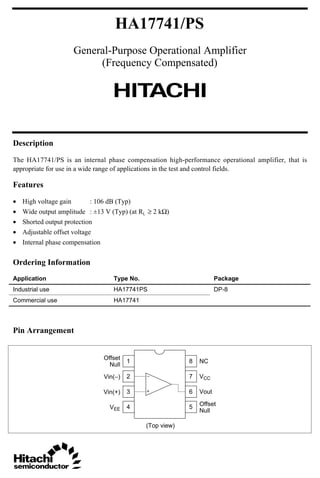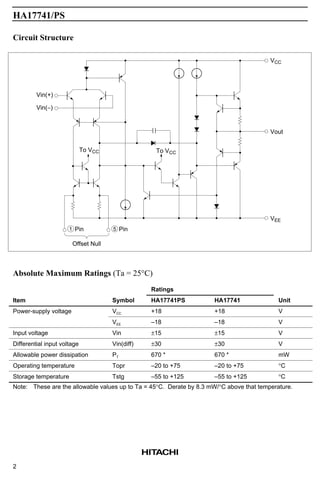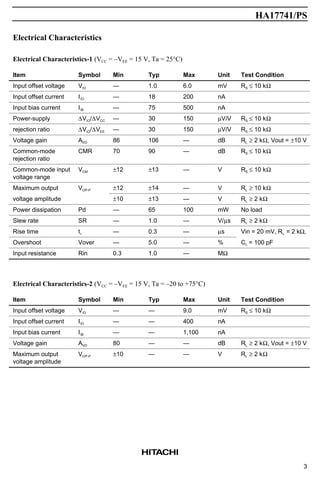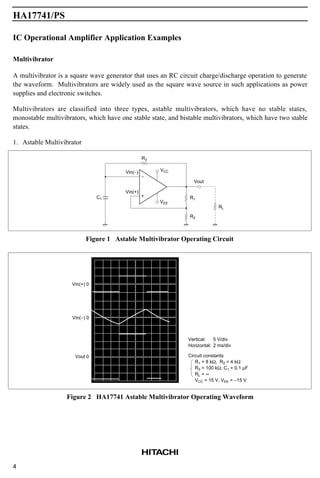Anúncio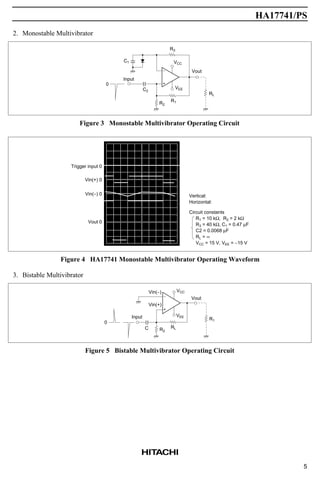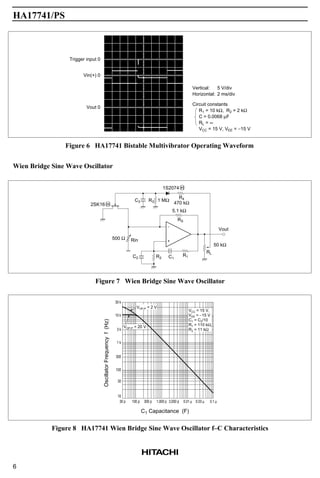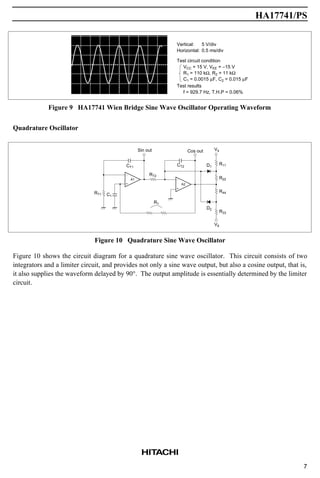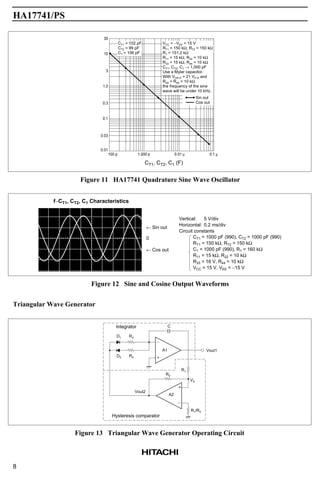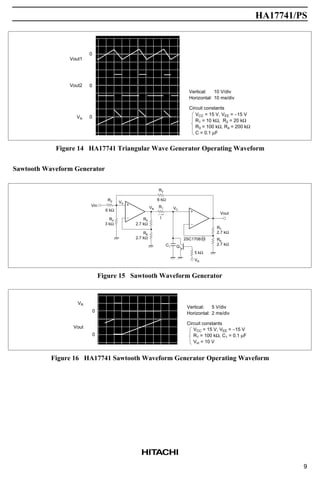Anúncio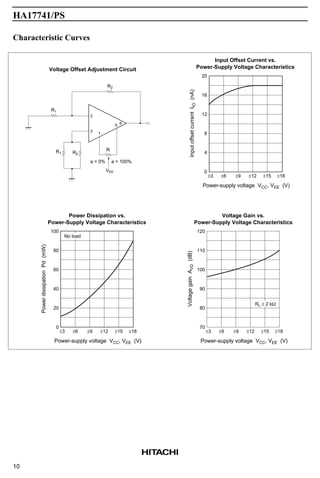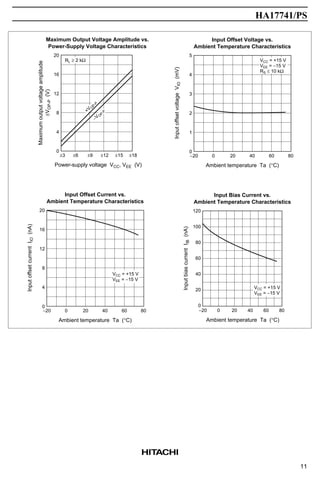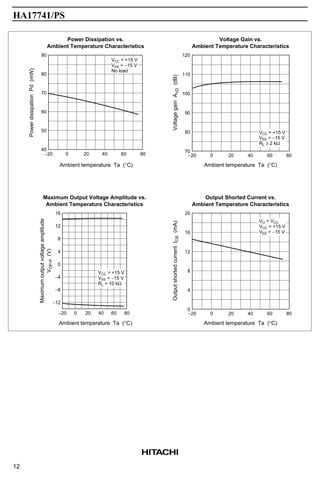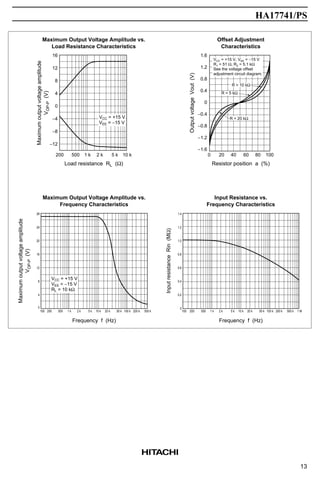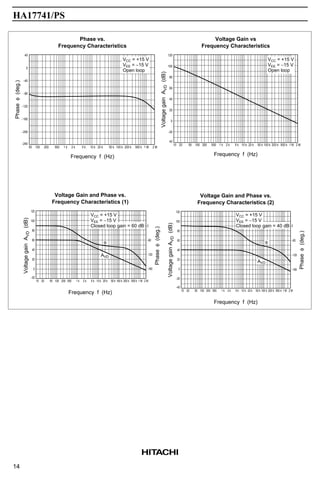Anúncio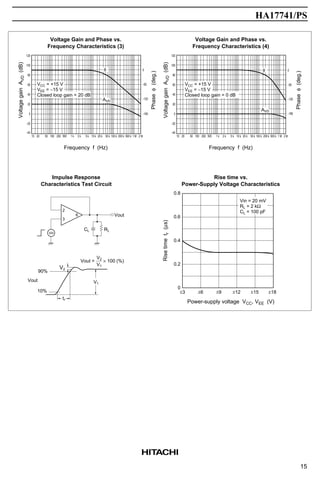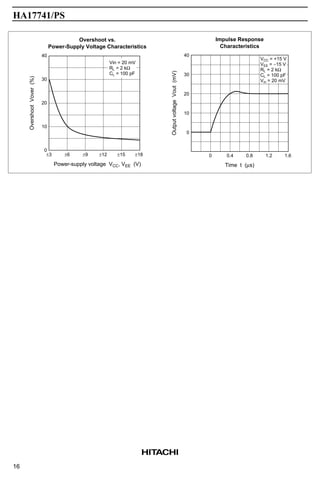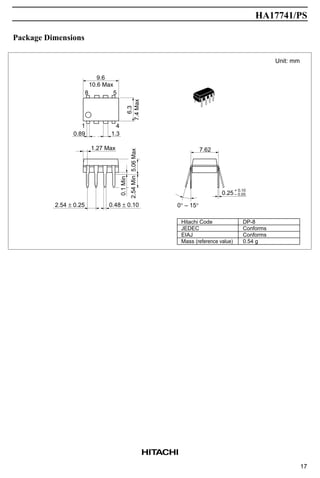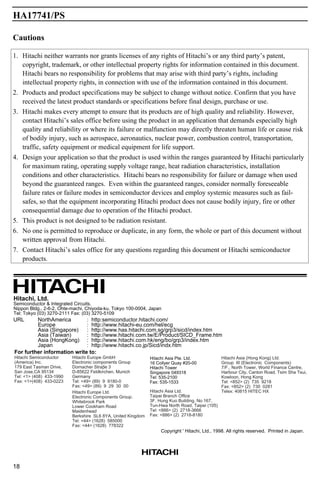Próximos SlideShares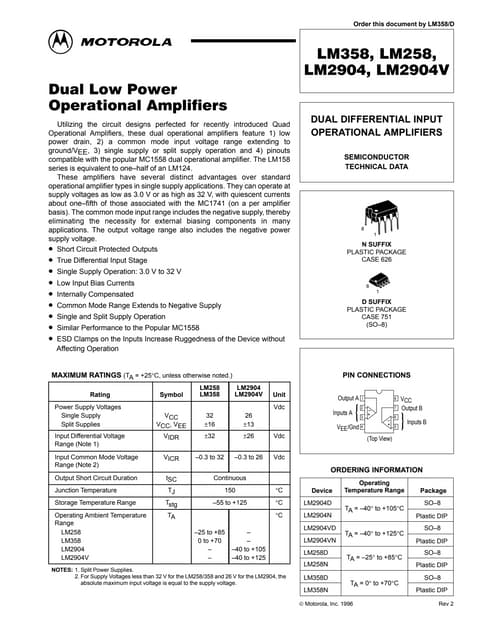Datasheet lm358
Carregando em ... 3
1 de 19
Anúncio

### HA17741 General Purpose Operational Amplifier

1. HA17741/PS General-Purpose Operational Amplifier (Frequency Compensated) Description The HA17741/PS is an internal phase compensation high-performance operational amplifier, that is appropriate for use in a wide range of applications in the test and control fields. Features • High voltage gain : 106 dB (Typ) • Wide output amplitude : ±13 V (Typ) (at RL ≥ 2 kΩ) • Shorted output protection • Adjustable offset voltage • Internal phase compensation Ordering Information Application Type No. Package Industrial use HA17741PS DP-8 Commercial use HA17741 Pin Arrangement − + 1 2 3 4 8 7 6 5 NC VCC Vout Offset Null Offset Null Vin(−) Vin(+) VEE (Top view)
2. HA17741/PS 2 Circuit Structure VCC Vout VEE To VCCTo VCC Offset Null Vin(+) Vin(−) Pin5Pin1 Absolute Maximum Ratings (Ta = 25°C) Ratings Item Symbol HA17741PS HA17741 Unit Power-supply voltage VCC +18 +18 V VEE –18 –18 V Input voltage Vin ±15 ±15 V Differential input voltage Vin(diff) ±30 ±30 V Allowable power dissipation PT 670 * 670 * mW Operating temperature Topr –20 to +75 –20 to +75 °C Storage temperature Tstg –55 to +125 –55 to +125 °C Note: These are the allowable values up to Ta = 45°C. Derate by 8.3 mW/°C above that temperature.
3. HA17741/PS 3 Electrical Characteristics Electrical Characteristics-1 (VCC = –VEE = 15 V, Ta = 25°C) Item Symbol Min Typ Max Unit Test Condition Input offset voltage VIO — 1.0 6.0 mV RS ≤ 10 kΩ Input offset current IIO — 18 200 nA Input bias current IIB — 75 500 nA Power-supply ∆VIO/∆VCC — 30 150 µV/V RS ≤ 10 kΩ rejection ratio ∆VIO/∆VEE — 30 150 µV/V RS ≤ 10 kΩ Voltage gain AVD 86 106 — dB RL ≥ 2 kΩ, Vout = ±10 V Common-mode rejection ratio CMR 70 90 — dB RS ≤ 10 kΩ Common-mode input voltage range VCM ±12 ±13 — V RS ≤ 10 kΩ Maximum output VOP-P ±12 ±14 — V RL ≥ 10 kΩ voltage amplitude ±10 ±13 — V RL ≥ 2 kΩ Power dissipation Pd — 65 100 mW No load Slew rate SR — 1.0 — V/µs RL ≥ 2 kΩ Rise time tr — 0.3 — µs Vin = 20 mV, RL = 2 kΩ, Overshoot Vover — 5.0 — % CL = 100 pF Input resistance Rin 0.3 1.0 — MΩ Electrical Characteristics-2 (VCC = –VEE = 15 V, Ta = –20 to +75°C) Item Symbol Min Typ Max Unit Test Condition Input offset voltage VIO — — 9.0 mV RS ≤ 10 kΩ Input offset current IIO — — 400 nA Input bias current IIB — — 1,100 nA Voltage gain AVD 80 — — dB RL ≥ 2 kΩ, Vout = ±10 V Maximum output voltage amplitude VOP-P ±10 — — V RL ≥ 2 kΩ
4. HA17741/PS 4 IC Operational Amplifier Application Examples Multivibrator A multivibrator is a square wave generator that uses an RC circuit charge/discharge operation to generate the waveform. Multivibrators are widely used as the square wave source in such applications as power supplies and electronic switches. Multivibrators are classified into three types, astable multivibrators, which have no stable states, monostable multivibrators, which have one stable state, and bistable multivibrators, which have two stable states. 1. Astable Multivibrator − + R3 VCC VEE Vout R1 R2 RL Vin(−) Vin(+) C1 Figure 1 Astable Multivibrator Operating Circuit Vin(+) 0 Vin(−) 0 Vout 0 Vertical: Horizontal: Circuit constants R1 = 8 kΩ, R2 = 4 kΩ R3 = 100 kΩ, C1 = 0.1 µF RL = ∞ VCC = 15 V, VEE = −15 V 5 V/div 2 ms/div Figure 2 HA17741 Astable Multivibrator Operating Waveform
5. HA17741/PS 5 2. Monostable Multivibrator − + R3 VCC VEE Vout RL R1R2 C2 C1 Input 0 Figure 3 Monostable Multivibrator Operating Circuit Vertical: Horizontal: Circuit constants R1 = 10 kΩ, R2 = 2 kΩ R3 = 40 kΩ, C1 = 0.47 µF C2 = 0.0068 µF RL = ∞ VCC = 15 V, VEE = −15 V Trigger input 0 Vin(+) 0 Vin(−) 0 Vout 0 Figure 4 HA17741 Monostable Multivibrator Operating Waveform 3. Bistable Multivibrator − + Vin(−) Vin(+) Input 0 C R2 RL VEE VCC R1 Vout Figure 5 Bistable Multivibrator Operating Circuit
6. HA17741/PS 6 Trigger input 0 Vin(+) 0 Vout 0 Vertical: Horizontal: Circuit constants 5 V/div 2 ms/div R1 = 10 kΩ, R2 = 2 kΩ C = 0.0068 µF RL = ∞ VCC = 15 V, VEE = −15 V Figure 6 HA17741 Bistable Multivibrator Operating Waveform Wien Bridge Sine Wave Oscillator − + R4 470 kΩ 1 MΩ 1S2074 H R3C3 2SK16 H 500 Ω Rin C2 R2 C1 R1 5.1 kΩ RS RL 50 kΩ Vout Figure 7 Wien Bridge Sine Wave Oscillator VCC = 15 V, VEE = −15 V C1 = C2/10 R1 = 110 kΩ, R2 = 11 kΩ VOP-P = 2 V VOP-P = 20 V 30 k 10 k 3 k 1 k 300 100 30 10 30 p 100 p 300 p 1,000 p 3,000 p 0.01 µ 0.03 µ 0.1 µ C1 Capacitance (F) OscillatorFrequencyf(Hz) Figure 8 HA17741 Wien Bridge Sine Wave Oscillator f–C Characteristics
7. HA17741/PS 7 Vertical: Horizontal: Test circuit condition 5 V/div 0.5 ms/div VCC = 15 V, VEE = −15 V R1 = 110 kΩ, R2 = 11 kΩ C1 = 0.0015 µF, C2 = 0.015 µF Test results f = 929.7 Hz, T.H.P = 0.06% Figure 9 HA17741 Wien Bridge Sine Wave Oscillator Operating Waveform Quadrature Oscillator − + A2 − + A1 V4 R11 R22 R44 R33 V8 D1 D2 Cos outSin out CT2 RT2 CT1 RT1 C1 R1 Figure 10 Quadrature Sine Wave Oscillator Figure 10 shows the circuit diagram for a quadrature sine wave oscillator. This circuit consists of two integrators and a limiter circuit, and provides not only a sine wave output, but also a cosine output, that is, it also supplies the waveform delayed by 90°. The output amplitude is essentially determined by the limiter circuit.
8. HA17741/PS 8 30 10 CT1 = 102 pF CT2 = 99 pF C1 = 106 pF VCC = −VEE = 15 V RT1 = 150 kΩ, RT2 = 150 kΩ R1 = 151.2 kΩ R11 = 15 kΩ, R22 = 10 kΩ R33 = 15 kΩ, R44 = 10 kΩ CT1, CT2, C1 → 1,000 pF Use a Mylar capacitor. With VOP-P = 21 VP-P and R22 = R44 = 10 kΩ the frequency of the sine wave will be under 10 kHz. Sin out Cos out 3 1.0 0.3 0.1 0.03 0.01 100 p 1,000 p 0.01 µ 0.1 µ CT1, CT2, C1 (F) Figure 11 HA17741 Quadrature Sine Wave Oscillator f−CT1, CT2, C1 Characteristics Vertical: Horizontal: Circuit constants 5 V/div 0.2 ms/div CT1 = 1000 pF (990), CT2 = 1000 pF (990) RT1 = 150 kΩ, RT2 = 150 kΩ C1 = 1000 pF (990), R1 = 160 kΩ R11 = 15 kΩ, R22 = 10 kΩ R33 = 16 V, R44 = 10 kΩ VCC = 15 V, VEE = −15 V ← Sin out 0 ← Cos out Figure 12 Sine and Cosine Output Waveforms Triangular Wave Generator − + A1 − + A2 D1 R3 D2 R4 C R1 R2 Vout2 VA R1/R2 Vout1 Hysteresis comparator Integrator Figure 13 Triangular Wave Generator Operating Circuit
9. HA17741/PS 9 Vertical: Horizontal: Circuit constants 10 V/div 10 ms/div VCC = 15 V, VEE = −15 V R1 = 10 kΩ, R2 = 20 kΩ R3 = 100 kΩ, R4 = 200 kΩ C = 0.1 µF 0 0 0 Vout1 Vout2 VA Figure 14 HA17741 Triangular Wave Generator Operating Waveform Sawtooth Waveform Generator + − + − Vin R2 6 kΩ VA R4 3 kΩ VB R3 6 kΩ R1 IR5 2.7 kΩ R6 2.7 kΩ C1 Q1 VR 5 kΩ 2SC1706 H Vout R7 2.7 kΩ R8 2.7 kΩ VC Figure 15 Sawtooth Waveform Generator 0 0 VR Vout Vertical: Horizontal: Circuit constants 5 V/div 2 ms/div VCC = 15 V, VEE = −15 V R1 = 100 kΩ, C1 = 0.1 µF Vin = 10 V Figure 16 HA17741 Sawtooth Waveform Generator Operating Waveform
10. HA17741/PS 10 Characteristic Curves 2 3 1 5 6 ±3 ±6 ±12 ±15 20 16 12 8 4 0 InputoffsetcurrentIIO(nA) Power-supply voltage VCC, VEE (V) Input Offset Current vs. Power-Supply Voltage Characteristics ±9 ±18 R1 R2 R2 R1 R a = 100%a = 0% VEE Voltage Offset Adjustment Circuit ±3 ±6 ±12 ±15 100 80 60 40 20 0 PowerdissipationPd(mW) Power-supply voltage VCC, VEE (V) Power Dissipation vs. Power-Supply Voltage Characteristics ±9 ±18 ±3 ±6 ±12 ±15 120 110 100 90 80 70 VoltagegainAVD(dB) Power-supply voltage VCC, VEE (V) Voltage Gain vs. Power-Supply Voltage Characteristics ±9 ±18 RL ≥ 2 kΩ No load
11. HA17741/PS 11 ±3 ±6 ±12 ±15 20 16 12 8 4 0 Maximumoutputvoltageamplitude ±VOP-P(V) Power-supply voltage VCC, VEE (V) Maximum Output Voltage Amplitude vs. Power-Supply Voltage Characteristics ±9 ±18 −20 0 20 40 60 5 4 3 2 1 0 InputoffsetvoltageVIO(mV) Ambient temperature Ta (°C) Input Offset Voltage vs. Ambient Temperature Characteristics 80 VCC = +15 V VEE = −15 V RS ≤ 10 kΩ RL ≥ 2 kΩ −V O P-P +V O P-P −20 0 20 40 60 20 16 12 8 4 0 InputoffsetcurrentIIO(nA) Ambient temperature Ta (°C) Input Offset Current vs. Ambient Temperature Characteristics 80 −20 0 20 40 60 120 100 80 60 40 20 0 InputbiascurrentIIB(nA) Ambient temperature Ta (°C) Input Bias Current vs. Ambient Temperature Characteristics 80 VCC = +15 V VEE = −15 V VCC = +15 V VEE = −15 V
12. HA17741/PS 12 −20 0 20 40 60 120 110 100 90 80 70 VoltagegainAVD(dB) Ambient temperature Ta (°C) Voltage Gain vs. Ambient Temperature Characteristics 80−20 0 20 40 60 90 80 70 60 50 40 PowerdissipationPd(mW) Power Dissipation vs. Ambient Temperature Characteristics 80 Ambient temperature Ta (°C) VCC = +15 V VEE = −15 V No load VCC = +15 V VEE = −15 V RL ≥ 2 kΩ −20 0 20 40 60 20 16 12 8 4 0 OutputshortedcurrentIOS(mA) Ambient temperature Ta (°C) Output Shorted Current vs. Ambient Temperature Characteristics 80−20 0 40 60 16 12 8 4 0 −4 −8 −12 Maximumoutputvoltageamplitude VOP-P(V) Maximum Output Voltage Amplitude vs. Ambient Temperature Characteristics 20 80 Ambient temperature Ta (°C) VO = VCC VCC = +15 V VEE = −15 V VCC = +15 V VEE = −15 V RL = 10 kΩ
13. HA17741/PS 13 200 500 1 k 2 k 5 k 16 12 8 4 0 −4 −8 −12 Maximumoutputvoltageamplitude VOP-P(V) Maximumoutputvoltageamplitude VOP-P(V) Maximum Output Voltage Amplitude vs. Load Resistance Characteristics 10 k Load resistance RL (Ω) 0 1.6 1.2 0.8 0.4 0 −0.4 −0.8 −1.2 −1.6 OutputvoltageVout(V) 20 40 60 80 100 VCC = +15 V VEE = −15 V VCC = +15 V, VEE = −15 V R1 = 51 Ω, R2 = 5.1 kΩ See the voltage offset adjustment circuit diagram. Offset Adjustment Characteristics Resistor position a (%) R = 10 kΩ R = 5 kΩ R = 20 kΩ 500 1 k 50 k 100 k 28 24 20 16 12 8 4 Frequency f (Hz) Maximum Output Voltage Amplitude vs. Frequency Characteristics 200 2 k 5 k 10 k 20 k 200 k 500 k 100 500 1 k 50 k 100 k 1.4 1.2 1.0 0.8 0.6 0.4 0.2 0 InputresistanceRin(MΩ) Frequency f (Hz) Input Resistance vs. Frequency Characteristics 200 2 k 5 k 10 k 20 k 200 k 500 k 1 M 0 VCC = +15 V VEE = −15 V RL = 10 kΩ 100
14. HA17741/PS 14 50 200 1 k 50 k 100 k 40 0 −40 −80 −120 −160 −200 Phaseφ(deg.) Frequency f (Hz) Phase vs. Frequency Characteristics 100 2 k 5 k 10 k 20 k 200 k 500 k −240 500 1 M 2 M 10 50 200 10 k 20 k 120 100 80 60 40 20 0 −20 VoltagegainAVD(dB) Frequency f (Hz) Voltage Gain vs Frequency Characteristics 20 500 1 k 2 k 5 k 50 k 100 k 40 100 500 k 2 M200 k 1 M VCC = +15 V VEE = −15 V Open loop VCC = +15 V VEE = −15 V Open loop 10 50 200 10 k 20 k 120 100 80 60 40 20 0 VoltagegainAVD(dB) Frequency f (Hz) Voltage Gain and Phase vs. Frequency Characteristics (1) 20 500 1 k 2 k 5 k 50 k 100 k −20 100 200 k 500 k 1 M 2 M 10 50 200 10 k 20 k 120 100 80 60 40 20 0 −20 VoltagegainAVD(dB) Frequency f (Hz) Voltage Gain and Phase vs. Frequency Characteristics (2) 20 500 1 k 2 k 5 k 50 k 100 k −40 100 200 k 500 k 1 M 2 M VCC = +15 V VEE = −15 V Closed loop gain = 60 dB VCC = +15 V VEE = −15 V Closed loop gain = 40 dB0 −60 −120 −180 0 −60 −120 −180 Phaseφ(deg.) Phaseφ(deg.) AVD φ φ AVD
15. HA17741/PS 15 10 50 200 10 k 20 k 120 100 80 60 40 20 0 −20 VoltagegainAVD(dB) Frequency f (Hz) Voltage Gain and Phase vs. Frequency Characteristics (3) 20 500 1 k 2 k 5 k 50 k 100 k100 200 k 500 k 1 M 2 M 0 −60 −120 −180 Phaseφ(deg.) −40 VCC = +15 V VEE = −15 V Closed loop gain = 20 dB AVD φ 10 50 200 10 k 20 k 120 100 80 60 40 20 0 −20 VoltagegainAVD(dB) Frequency f (Hz) Voltage Gain and Phase vs. Frequency Characteristics (4) 20 500 1 k 2 k 5 k 50 k 100 k100 200 k 500 k 1 M 2 M 0 −60 −120 −180 Phaseφ(deg.) −40 VCC = +15 V VEE = −15 V Closed loop gain = 0 dB AVD φ 2 3 6 ±3 ±6 ±9 ±12 ±15 0.8 0.6 0.4 0.2 0 Risetimetr(µs) Power-supply voltage VCC, VEE (V) Rise time vs. Power-Supply Voltage Characteristics ±18 Impulse Response Characteristics Test Circuit Vout RLCL V2 90% 10% Vout tr V1 Vout = × 100 (%) V2 V1 Vin = 20 mV RL = 2 kΩ CL = 100 pF Vin
16. HA17741/PS 16 ±3 ±6 ±9 ±12 ±15 40 30 20 10 0 OvershootVover(%) Power-supply voltage VCC, VEE (V) Overshoot vs. Power-Supply Voltage Characteristics ±18 0 0.4 0.8 1.2 40 30 20 10 0 OutputvoltageVout(mV) Time t (µs) Impulse Response Characteristics 1.6 Vin = 20 mV RL = 2 kΩ CL = 100 pF VCC = +15 V VEE = −15 V RL = 2 kΩ CL = 100 pF Vin = 20 mV
17. HA17741/PS 17 Package Dimensions Hitachi Code JEDEC EIAJ Mass (reference value) DP-8 Conforms Conforms 0.54 g Unit: mm 1 4 58 9.6 10.6 Max 0.89 1.3 6.3 7.4Max 2.54Min5.06Max 2.54 ± 0.25 0.48 ± 0.10 7.62 0.25 + 0.10 – 0.05 0° – 15° 0.1Min 1.27 Max